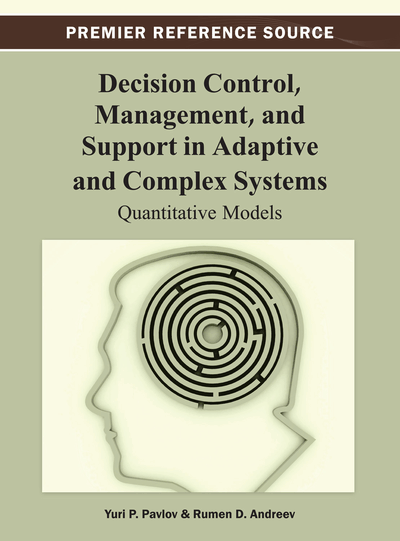# Elements of Stochastic Programming

DOI: 10.4018/978-1-4666-2967-7.ch005

## Abstract

In the second part of the chapter is discussed the “potential functions method,” a very fruitful area of the stochastic programming. Even though it is called method, this field is actually a mathematical theory whose practical results are a large number of stochastic recurrent procedures for pattern recognition, approximating algorithms for functions in noisy conditions, development of unified mathematical approach for machine learning on the basis of human preference, proof of the perceptron theory, etc. The stochastic algorithms based on the “potential functions method” have stable convergence and flexibility, and these properties permit fruitful application in utility and value function evaluations and polynomial approximations. The last part of the chapter gives an example of pattern recognition of two sets of positive and negative answers as machine learning procedure.
Chapter Preview
Top

## 1. Beginnings: Stochastic Programming

The stochastic programming is a field in the theory of optimal decisions, in which problems for determining optimal choice in conditions described with random functions are studied (Aizerman, 1970; Ermolev, 1976), or in other words, this is a theory for solving extremal problems of stochastic nature. In this book, we will use mathematical techniques for solving problems from the decision-making theory and analytical approximation of value, utility functions and subjective probabilities, whose existence in axiomatic aspect is analyzed in the theory of measurement and the utility theory (Pfanzagl, 1971; Keneey, 1993). In the previous two chapters, we looked into some axiomatic systems and existence theorems regarding these functions and we discussed the uncertainty, which occurs in the practical aspects of the real-world decision-making problems on the basis of the normative approach. We saw that the human preferences, which by theoretical formulation are fundamental for the determination of the value and utility functions, are characterized in their explicit expression by uncertainty of stochastic nature (Keneey, 1993; Mengov, 2010). Consecutively exposing our preferences in the area of the specific problem to be solved, we gradually provide increasing details for our attitude in recurrent manner. Because of this, the problems and methods of stochastic programming of recurrent nature are of great interest to us (Pavlov, 1989, 2003, 2011). Such are the quasi-gradient recurrent methods to which we will limit our considerations and which we will discuss in more details for the purpose of this book (Robbins, 1951; Aizerman, 1970; Ermolev, 1976).

The gradient of a nonlinear function F(x1, x2, …,xn),cannot be precisely determined in the stochastic programming, when the function is given algorithmically. Because of this in the stochastic programming problems a quasi-gradient is introduced, a random vector whose mathematical expectation is close to the gradient or to the generalized gradient of the investigated functions (see paragraph 2.2). Let us want to minimize the convex function:

F(x1, x2, …, xn), (x1, x2, …,xn)∈X.

In the problems, we suppose that the set X is convex and closed. We already mentioned that with respect to the generalized gradient we can only have statistical estimates of random vectors due to the algorithmic determination of the objective function. When the set X is bounded, in the stochastic recurrent quasi-gradient procedures the prjection π(z) may be used. For every point z, z∈Rn, the point πX(z) belongs to X, πX(z)X and hence:

y - πX(z)║≤ ║y - z║, ∀yX.

## Complete Chapter List

Search this Book:
Reset